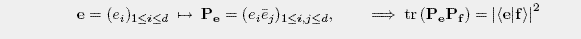Gerhard Zauner

MUBs (Mutually Unbiased Bases) and SIC POVMs (Symmetric Informationally Complete Positive Operator Valued Measures) are both regular quantum designs with r=1. Such designs are also called spherical designs, as they can be described using normalized vektors e• MUBs
are regular (r=1) and affine quantum designs. In the vector description the orthogonal classes are orthogonal bases, were for vectors e, f from different bases one gets |<e|f>|2=1/d.
The maximum number of MUBs is d+1. Sets of d+1 MUBs when d is a prime were first found by I.D.Ivanovic (1981). W.K.Wooters and B.D.Fields established 1989 the term "mutually unbiased" and gave solutions of d+1 MUBs whenever d is a power of a prime.
In my master thesis (1991) this concept was independently rediscovered and generalized to irregular designs (independent observables, see next page). Furthermore maximal solutions were found the for more general concept of affine quantum designs with r(d2-1)/(d-r) complete orthogonal classes whenever d is a powers of a prime. For d=6 an infinite family of MUB-triples was constructed and it was (probably the first time) conjectured that 4 MUBs don't exist in this dimension.
For a recent overview see T.Durt, B.G.Englert, I.Bengtsson, K.Zyczkowski arXiv:1004.3348 (external link).

• SIC POVMs
are regular (r=1) quantum designs with degree 1 and d2 elements. For any two different vectors e, f the equation <e|f>|2=1/(d+1) holds. Thus the vectors span d2 equiangular lines.
Equiangular lines were first investigated by J.J.Seidel (1966, 1973, ...) et.al., mainly for the real case. S.G.Hoggar (1982) analyzed the complex case and gave solutions (of d2 vectors) for d=2,3 and 8.
In my Ph.D. thesis (1999) further maximal analytic and numerical solutions weren given and it was (probably the first time) conjectured that such solutions in the complex case exist in any dimension d (generated by the Weyl-Heisenberg group and with a certain additional symmetry of order 3). See also Problems in Quantum Information Theory, Nr.23 (external link).
J.M.Renes, R.Blume-Kohout, A.J.Scott and C.M.Caves independently analyzed the same structures in 2003, gave further numerical solutions and coined the term SIC-POVM.
For a recent overview about know solutions see A.J.Scott, M.Grassl arXiv:0910.5784 (external link).

It can be shown, that both, sets of d+1 MUBs (more generally affine quantum designs with r(d2-1)/(d-r) complete orthogonal classes) and SIC-POVMs (more generally regular quantum designs with degree 1 and d2 elements) are quantum 2-design with respect to the group G = U(d), of all unitary matrices.
 Dieser Text ist nur auf Englisch verfügbar